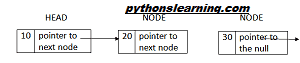Reverse A Linked List Hackerrank Solution Python

## Reverse a linked list hackerrank solution python

Reversed list 7->84->23->24->12
A linked list is a data structure which is made of a chain of node objects. Each
node consist of a value and a pointer. Pointer(memory location) which is link to
the next node in the chain.

In linked list we can perform insertion, deletion operation too.
The head pointer points to the first node of the list, and the last element of the
list points to null. When the list is empty, the head pointer points to null.

``````HEAD NODE NODE
10 pointer to
next node

2
0
pointer to
next node

3
0
pointer to
the null

null``````

The first thing to create a class for the nodes. The objects of this class will be the
nodes that we will insert in our linked list. The node for the linked list contains the
data and the pointer(memory location) to the next node. So, node class will
contain two variables that is data and next. The value of data is set through the
constructor code.

``````class Node:
def __init__(self, data=None, next=None):
self.data = data
self.next = next

2``````

create the class for the Linked List. in this class we can insert,delete,traverse and
sort the list first the class contain only one node which contain one node that is
head node that point to the first node. The value of head node to be null using
the constructor while . The following script creates a class for the linked list.

``````class LinkedList:
def __init__(self):
self.start_node = None
``````

Now we have created a class for our single list. The next step is to add insertion
function to insert items into the linked list.
Inserting element in the linked list is the process of reassign the pointer from the
existing to the newly inserted node. It all based on the position of insertion that is
we can insert the new node to the beginning , last , middle of the list.

``````def insert(self, data):
newNode = Node(data)
while (current.next):
current = current.next
current.next = newNode
else:
Then add the print function to print the node of the linked
list.
def printLL(self):
while (current):
print(current.data)
current = current.next

3``````

then add the reverse function to reverse the list .First create before variable
which is initially set as none.the make the head node as current and the first node
of the linked list is next.This method repeat till the last node of the list.

``````ef reverseList(list):
before = None
next = current.next
while current:
current.next = before
before = current
current = next
if next:
next = next.next

Then add the driver code input from the user by making range for no.of element
for the list. Then add all element to the linked list and call the reverse function
and print that.

``````LL = LinkedList()
n=int(input(&quot;Enter the no.of element for linked list:&quot;))
i=0
for i in range(n):
a=int(input(&quot;enter the element&quot;))
LL.insert(a)
i = 1 + 1
LL.printLL()
reverseList(LL)
LL.printLL()``````

Summary :

K.Kathir oli
2nd year B.tech Cse